# Deep Learning — Week 4

Moving on from autoencoders, it’s time to look at the most widely used applications of Deep Learning. If you missed the previous article on autoencoders, you can find it here: https://bit.ly/3c2nxNv

Neural networks are known for their mechanism on extracting hidden patterns; patterns that cannot be distinctly observed by the human eye as well. This property of a neural network can come in handy for recognising patterns in images and classifying the object inside the image accordingly. But before we go about classifying images, let’s look at how convolution works.

The Convolution Operation:

Consider a scenario wherein we’re tracking the position of an aeroplane using a laser sensor at regular intervals in the presence of noise.

In order to obtain a less noisy estimate we would like to take the average several measurements. And since the newer, most recent measurements are more important than the previous measurements, we’d go ahead with the weighted average.

The sum of all weighted values of x is known as a convolution.

The weights array ‘w’ is known as the filter or the kernel. In practice, we sum over a small window (filter) and slide the filter over the input and compute the value of ‘s’ based on a window around ‘x’.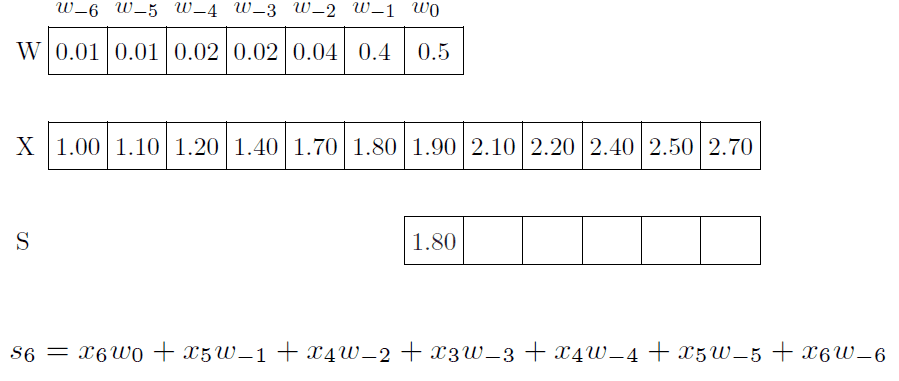Filter slides over the input vector to obtain the convolution sum.

Here, the input and the kernel both are one-dimensional. How does it look for two dimensions?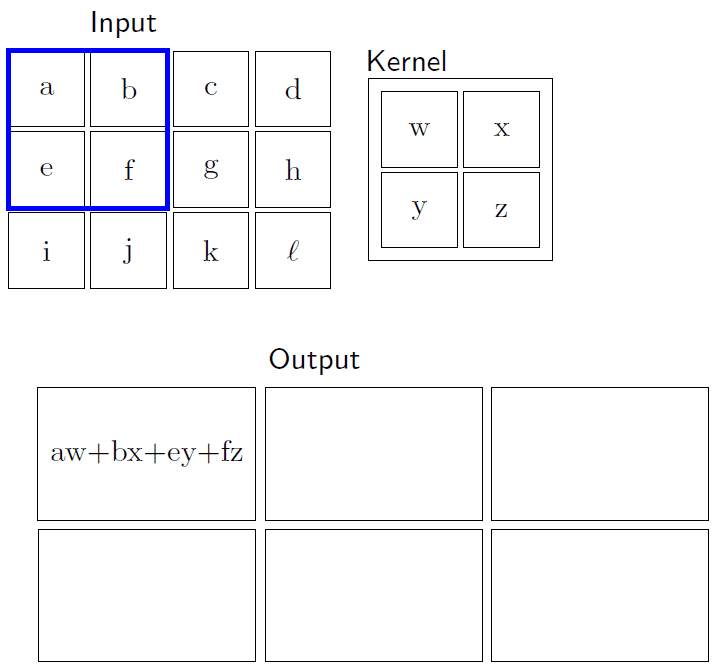Convolution with a 2D input matrix and a 2D kernel (filter).

For the rest of our discussion, we will consider the kernel to be centred at the pixel of interest.

So in essence, the kernel will overlap with the pixel’s preceding and succeeding neighbour pixels as well.

Some examples of 2D convolution applied to images is as follows:

So how does a 2D convolution work with images?

We just slide the kernel over the input image, and each time we slide the kernel we get one value in the output.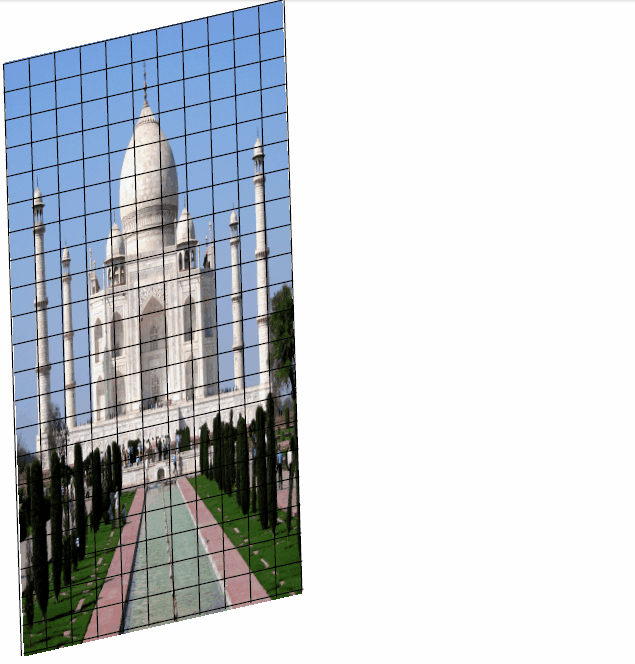Sliding the kernel over the input image results in an output of same dimensions but smaller length and width.

The resulting output is called a feature map, and multiple filters can be used to obtain multiple feature maps for an input image.

• A filter for 3D convolution will be in 3D and will be referred to as a volume.
• We slide the volume over the 3D input matrix and compute the convolution per step.
• In effect, we’re doing a 2D convolution operation on a 3D input as the filter slides along the length and breadth but not along its depth.
• As a result, the output of the convolution operation will be 2D.
(only width and height, no depth).
• As is the case with 2D convolution, we can apply multiple filters to get multiple feature maps with 3D input images as well.

Before moving on to Convolutional Neural Networks, it is essential to define the following quantities:

• ‘W1’ is the width of the input image.
• ‘H1’ is the height of the input image.
• ‘D1’ is the depth of the image (for a monochrome image, it’s 1 whereas for a coloured image it’s 3, with RGB channels).
• ‘S’ stands for stride, i.e. the number of pixels you shift the filter by while sliding it across the image.
• ‘K’ stands for the number of filters used.
• ‘F’ is the spatial extent of each filter (the depth of filter is the same as depth of the input).
• Output: W2 x H2 x D2, where D2 is the number of filters (K).

The output consists of all feature maps obtained from different filters stacked against each other. Hence, the depth of the output is equal to the number of feature maps/ number of kernels.

## Convolutional Neural Networks

Consider the task of simple Image Classification (recognising the contents of an image and assigning a certain label to them: for example, a picture of the Taj Mahal can be classified/ assigned the label of “monument”.)

In the earlier examples of applying kernels to images and performing convolutions, we observed that we used hand-crafted kernels such as edge detectors and sharpeners to extract features from images.

Instead of using handcrafted kernels, can we let the model decide on the best kernels for a given input image? Can we enable the model to learn multiple kernels on its own, in addition to learning the weights of the classifier?

Convolutional Neural Networks aim to achieve the exact same goal. Kernels can be treated as parameters and learnt in addition to the weights of the classifier, using backpropagation.

But how is this different from a regular feed-forward neural network?

Consider the example in the network shown above. An image (4px * 4px) can be flattened into a linear array of 16 input nodes for a neural network.

We observe that there are a lot of dense connections, which not just lead to heavier computation, but also loss in consistency of extracted features.

Contrast this to the case of convolution.

Convolution takes advantage of the structure of the image, as it is important to know that interactions between neighboring pixels are more interesting and significant for the determination of what the entire picture represents.

Moreover, convolution leads to sparse connectivity which reduces the number of parameters in the model.

But is sparse connectivity really a good thing? Aren’t we losing information by losing interaction between neighbouring input pixels?

• Well, not really. If anything, losing interaction can prove to be beneficial as the model progresses.
• Consider the case of neurons x1 and x5. They don’t interact with each other directly on layer 1.
• However, they happen to interact at layer 2, where their respective characteristics are more profound.

Weight Sharing is another advantage of Convolutional Neural Networks. Essentially, we can apply different kernels at all locations in an image and the kernels will be shared by all the locations. That way, the job of learning parameters (kernels) becomes more distributed and easier (instead of trying to learn the same weights / kernels at different locations again and again).

Here’s what a complete Convolutional Neural Network looks like: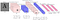A complete Convolutional Neural Network with alternating convolution and pooling layers.

What does a pooling layer do?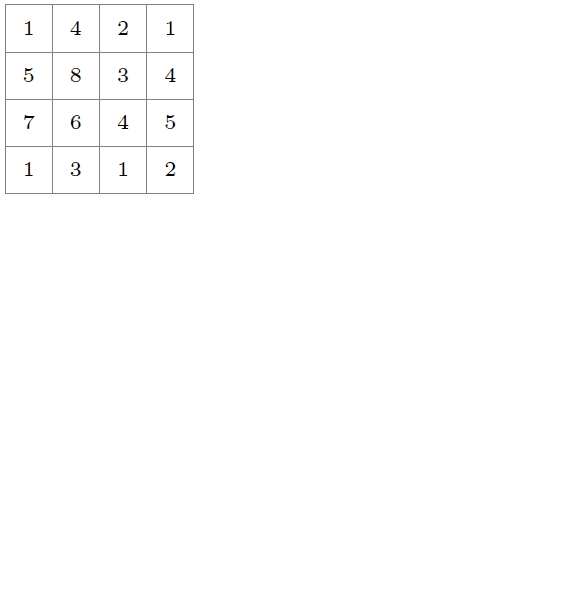Max-pooling takes the largest value covered by the filter over the feature map.

As shown in the GIF above, pooling generally reduces the size of feature map obtained after a convolution operation. Max-pooling generally includes the maximum value overlapped by the kernel in the output.

Average pooling takes the average of all values overlapped by the kernel.

How do we train a convolutional neural network?

A CNN can be trained as a regular feedforward neural network, wherein only a few weights are active (in colour).
The rest of the weights (in gray) are zero, and the final outcome is a neural network consisting of sparse connections.

Thus, we can train a convolutional neural network using backpropagation by thinking of it as a feedforward neural network with sparse connections.

Visualizing patches which maximally activate a neuron

• Consider some neurons in a given layer of a CNN.
• We can feed in images to this CNN and identify the images which cause these neurons to fire.
• We can then trace back to the patch in the image which causes these neurons to fire.
• In an experiment conducted in 2014, scientists considered neurons in the pool5 layer and found patches which caused the neurons to fire.
• One neuron fired for people’s faces
• One neuron fired for dog snouts
• Another fired for flowers, while another fired for flowers.

So how do we visualize filters in the first place?

Recall that we’d done something similar with autoencoders. We’re interested in finding an input which maximally excites a neuron.

Turns out, the input which will maximally activate a neuron is the normalized version of the filter, as per an optimization problem modelled as follows:

As mentioned earlier, we think of CNNs as feed-forward neural networks with sparse connections and weight sharing. Hence, the solution is the same here as well, since the parameter weights are nothing but the filters.

Thus, filters can be thought of as pattern detectors.

• Typically, we’re interested in understanding which portions of the image are responsible for maximizing the probability of a certain class.
• We could occlude (gray out) different patches in the image and see the effect on the predicted probability of the correct class.
• For example, these heatmaps show that occluding the main features of images result in huge drops in prediction probability.

So how can we gauge the influence of input pixels?

• We can think of images as grids of (m x n) pixels.
• We’re interested in finding the influence of each of these inputs(xi) on a given neuron(hj).
• In other words, we turn to gradients to understand the extent of dependency on certain input pixels.
• We could just compute partial derivatives of activation functions at a middle layer w.r.t. the input and visualize the gradient matrix as an image itself.

Hence, we can conclude:

Gradients can be computed by representing CNNs as feedforward neural networks and using backpropagation.

Computer Vision is a branch that thrives on the usage of Convolutional Neural Networks. There are many more concepts such as Guided Backpropagation, Deep Art, Deep fake and Deep dream among many others which are worth surfing the web for.

This issue marks the end of the Deep Learning month from FACE. We’ll be back next week with a new issue on a new domain. Stay tuned!

We are the departmental forum for Computer Science & Engineering, at Amrita School of Engineering , Bengaluru.

## More from FACE_ASEB

We are the departmental forum for Computer Science & Engineering, at Amrita School of Engineering , Bengaluru.